Like   Tweet   Pin
 /* styles */ Dear all, SPRING HAS SPRUNG but that's not all that's sprung up this spring! Contini Contemporary who represent my artwork in London and Italy just opened a new gallery.

## Contini Contemporary - Mayfair's Newest Art Gallery

 table div table+table+table+table div table td,table.module-3{width:100%;padding:0}table div table+table+table+table div table{width:100%;float:none;margin-left:auto;margin-right:auto;padding:0}table div table+table+table+table div table a{border:0 none;text-decoration:none}table div table+table+table+table div table img{width:100%!important;border:0 none;text-decoration:none}/* styles */
 table div table+table+table+table+table div table,table.module-4{width:48.11%;float:left;padding:0}table div table+table+table+table+table div table a{border:0 none;text-decoration:none}table div table+table+table+table+table div table img{width:100%!important;border:0 none;text-decoration:none}table div table+table+table+table+table div table td{width:100%;padding:0 20px 20px 0}/* styles */ The new gallery opened with a worldwide launch of "HashTag Queens" by "Robodrone", including live "Select Radio" broadcasting on Valentine's day: Contini Contemporary is now located at 15-16 Brook's Mews, Mayfair London W1K 4DS, Tel 020 3972 9001, email: info@continicontemporary.com. Now showing sculpture ‘PNUUU‘ 2019, a composition of three male back figures made from black finished steelmesh, height 53 cm. A short video shows different lighting of this artwork.
 table div table+table+table+table+table+table div table{width:100%;padding:0}table div table+table+table+table+table+table div table img{width:96.23%;padding:0;float:none}table div table+table+table+table+table+table div table td{width:100%;padding:0 1.88% 18px}/* styles */## Online: 1 Minute Sculpture Videos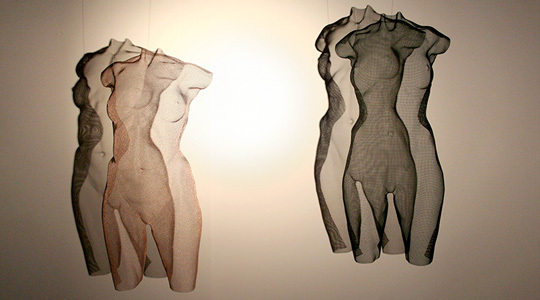Sculpture ’SENZ’ and ’SCIN’ with spotlights
 /* styles */ Some of my most recent works are featured in very short online videos to demonstrate the three-dimensional quality of the artworks. A simple spotlight produces dynamic results as you can see! Many thanks for the music by Jan Pulsford which I often listen to while sculpting at the studio.
 table div table+table+table+table+table+table+table+table+table+table+table div table{width:100%;padding:0}table div table+table+table+table+table+table+table+table+table+table+table div table img{width:96.23%;padding:0;float:none}table div table+table+table+table+table+table+table+table+table+table+table div table td{width:100%;padding:0 1.88% 18px}/* styles */## Solo Exhibitions David Begbie and Marta Jedrysko

 table div table+table+table+table+table+table+table+table+table+table+table+table+table div table td,table.module-12{width:100%;padding:0}table div table+table+table+table+table+table+table+table+table+table+table+table+table div table{width:100%;float:none;margin-left:auto;margin-right:auto;padding:0}table div table+table+table+table+table+table+table+table+table+table+table+table+table div table a{border:0 none;text-decoration:none}table div table+table+table+table+table+table+table+table+table+table+table+table+table div table img{width:100%!important;border:0 none;text-decoration:none}/* styles */
 /* styles */ Vught, The Netherlands, 30 March - 19 May 2019 Please join me at the opening event at Van Loon's superb gallery in central Holland on 30th March from 3pm if you can make it. The exhibition runs until late May so there is plenty of possibility to see a range of new sculptures like powerful torso sculpture ’TENZ’ 2019. I look forward to visiting the "David Begbie Room" and to seeing Marta's vivid paintings. Her way of painting is characterized by cheerful and bright colours and are juxtaposed with my monochrome sculpture in this double solo-show. More info on website Van Loon Galleries.
 table div table+table+table+table+table+table+table+table+table+table+table+table+table+table+table div table{width:100%;padding:0}table div table+table+table+table+table+table+table+table+table+table+table+table+table+table+table div table img{width:96.23%;padding:0;float:none}table div table+table+table+table+table+table+table+table+table+table+table+table+table+table+table div table td{width:100%;padding:0 1.88% 18px}/* styles */## Bel Air Fine Art Galleries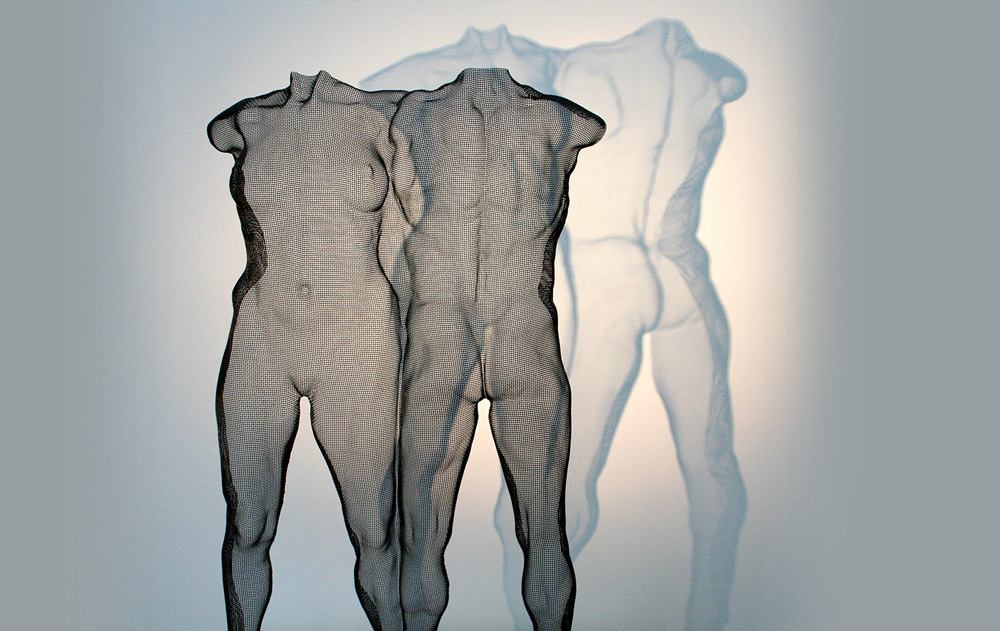Scupture composition ’DUO’ 2018, 75 x 48 x 15 cm, at Bel Air Fine Art Galleries
 /* styles */ Bel Air Fine Art Galleries are now exhibiting a selection of my work in Geneva, Crans-Montana & Gstaad, Switzerland, Knokke in Belgium as well as in Paris Rivoli and Cannes, France. François Chabanian's Bel Air Fine Art Galleries are already well established in towns and cities of France exhibiting international contemporary art of the highest caliber. François continues to expand his empire of galleries towards the rest of the world including London UK, the USA and the middle East and I am pleased to be part of his ambitious campaign.
 table div table+table+table+table+table+table+table+table+table+table+table+table+table+table+table+table+table+table+table div table{width:100%;padding:0}table div table+table+table+table+table+table+table+table+table+table+table+table+table+table+table+table+table+table+table div table img{width:96.23%;padding:0;float:none}table div table+table+table+table+table+table+table+table+table+table+table+table+table+table+table+table+table+table+table div table td{width:100%;padding:0 1.88% 18px}/* styles */## Frances Segelman's live-sculpting of Debbie Moore + Pinapple Dance Studios celebrates 40 yearsFrances Segelman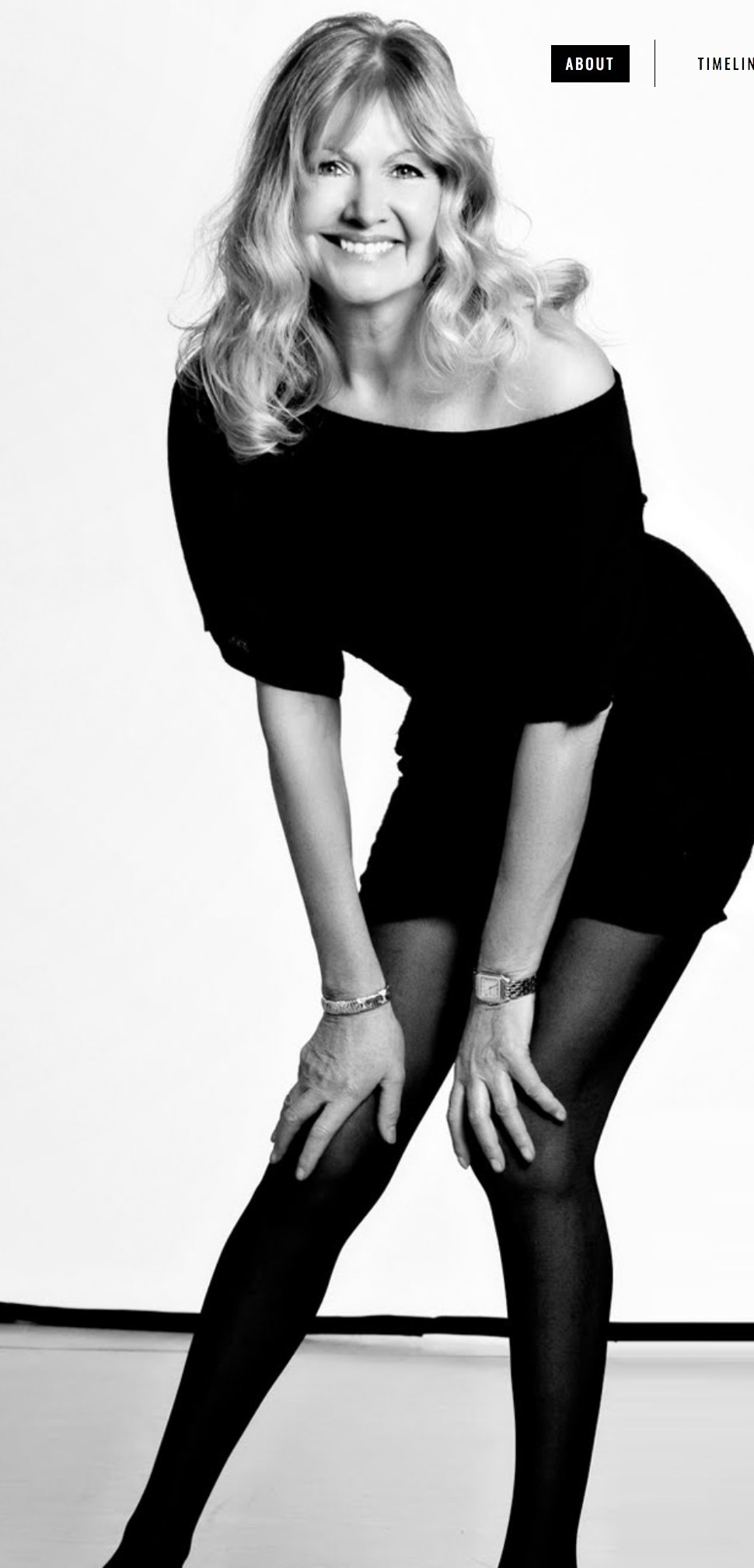Debbie Moore OBE
 /* styles */ I was kindly invited to exhibit ’XPOSE 1’ at this very special event and it was great fun to see Debbie Moore OBE, founder of Pineapple Dance Studios, being live-sculpted by Royal sculptor Frances Segelman on a great party night, marking Pineapple's 40th anniversary as well as the opening of a brand-new Pinapple Fashion shop. Debbie started her career at the age of 15, being the very first woman to list a company on the London Stock Exchange. Debbie founded Pinapple Dance Studios in 1979 in a disused pineapple warehouse in Covent Garden to make dance accessible to everyone. She also created the first versatile fabric for dance wear, used for bodies, leggings, dresses and skirts, creating the global Pineapple brand. Self-taught sculptress Frances Segelman has sculpted a wide variety of public figures including HM The Queen, HRH The Duke of Edinburgh and HRH The Prince of Wales and other VIP personalities. Other recent commissions include Lord Julian Fellowes, Dame Joanna Lumley and Dame Joan Collins. Congratulations to both from me!
 table div table+table+table+table+table+table+table+table+table+table+table+table+table+table+table+table+table+table+table+table+table+table+table div table{width:100%;padding:0}table div table+table+table+table+table+table+table+table+table+table+table+table+table+table+table+table+table+table+table+table+table+table+table div table img{width:96.23%;padding:0;float:none}table div table+table+table+table+table+table+table+table+table+table+table+table+table+table+table+table+table+table+table+table+table+table+table div table td{width:100%;padding:0 1.88% 18px}/* styles */## Hannah Peschar Sculpture Garden starts 29th March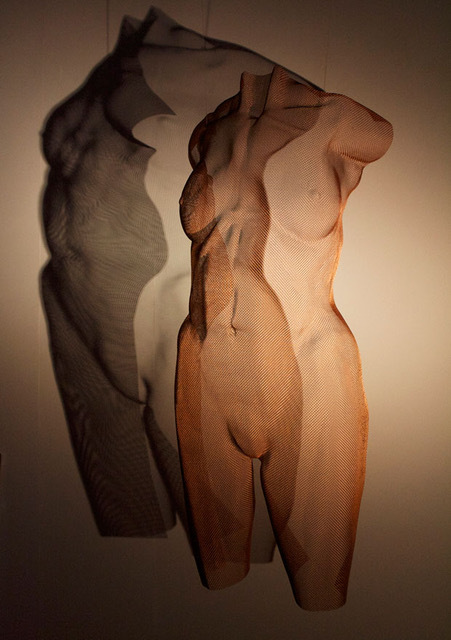‘VENUS‘ sculpture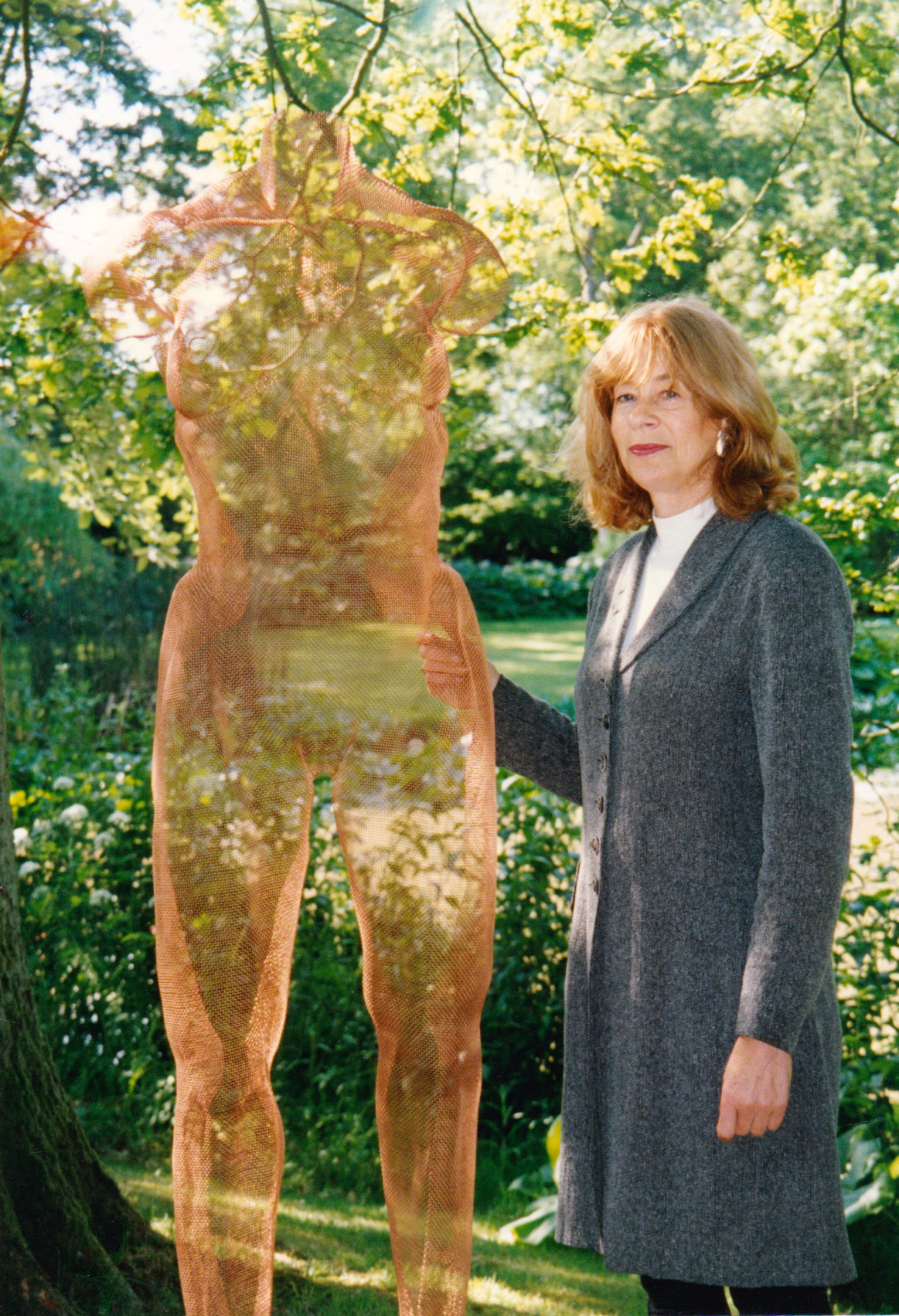Hannah Peschar in 2007

The new season at this wonderful sculpture garden starts very soon and I am pleased to exhibit here once again. Bronze-mesh sculpture 'ICONII' and 'VENUS' are normally shown indoors and I look forward to seeing them both in this fabulous outdoor setting at the Hannah Peschar Sculpture Garden, Surrey, curated by Anthony Paul and Vikki Leedham.
Please contact Vikki for an invitation to the forthcoming opening event and if you manage to get to the sculpture garden be prepared for a memorable day out!
All the best to Hannah who celebrated a round birthday recently!

 table div table+table+table+table+table+table+table+table+table+table+table+table+table+table+table+table+table+table+table+table+table+table+table+table+table+table+table div table{width:100%;padding:0}table div table+table+table+table+table+table+table+table+table+table+table+table+table+table+table+table+table+table+table+table+table+table+table+table+table+table+table div table img{width:96.23%;padding:0;float:none}table div table+table+table+table+table+table+table+table+table+table+table+table+table+table+table+table+table+table+table+table+table+table+table+table+table+table+table div table td{width:100%;padding:0 1.88% 18px}/* styles */## ‘Art Unbound‘ at the Rococo Garden, Painswick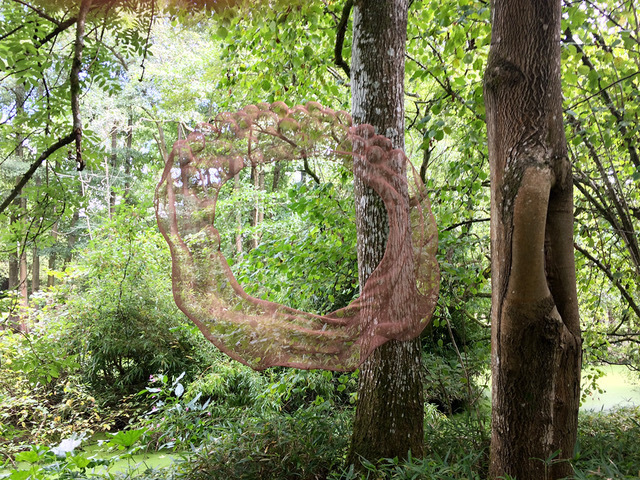Bronzemesh sculpture ‘SCRUMPLE‘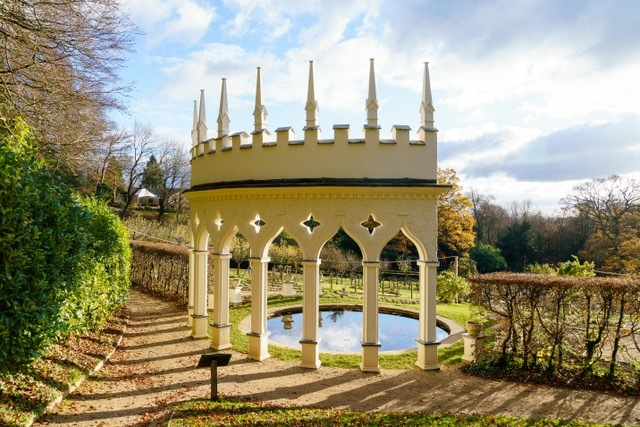Hidden gem: The Painswick Rococo Garden
 /* styles */ 26 May - 8 September 2019 Painswick Rococo Gardenwas designed as an English country gentleman's pleasure garden. Since 1984, the Garden has been rescued from ruin and transformed into a fanciful setting and is now the country's only complete surviving Rococo garden! Taking inspiration from the intentions of the garden, ‘Art Unbound‘ and curator Anna Greenacre from on Form at Asthall Manor in the Cotswolds bring together a selection of both established sculptors and new British talent working in wood, stone wire, ceramics and bronze to beautifully complement the design of the garden and attract more visitors to this hidden gem. I have chosen to exhibit sculptures in the Rococo spirit, mixing both abstract and figurative subjects which emphasis both classical and flamboyant decorative themes. ‘MYTHOS I and MYTHOS II‘ are male and female figures with drapery (in classical niches) and ‘SCRUMPLE‘ - a wreath of apples and drapery (at the apple press building) as well as ‘SOLN‘ (an angel sculpture at the Pigeon House), to mirror the playful spirit of the Rococo period. Here's the press release "Art Unbound" to download.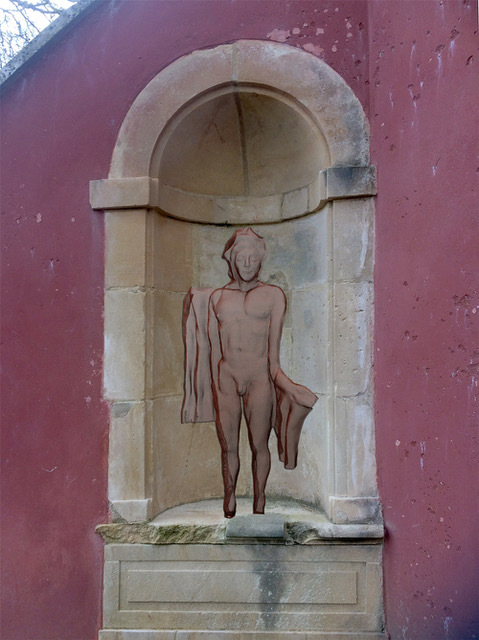‘MYTHOS I‘‘MYTHOS II‘
 table div table+table+table+table+table+table+table+table+table+table+table+table+table+table+table+table+table+table+table+table+table+table+table+table+table+table+table+table+table+table+table+table div table{width:100%;padding:0}table div table+table+table+table+table+table+table+table+table+table+table+table+table+table+table+table+table+table+table+table+table+table+table+table+table+table+table+table+table+table+table+table div table img{width:96.23%;padding:0;float:none}table div table+table+table+table+table+table+table+table+table+table+table+table+table+table+table+table+table+table+table+table+table+table+table+table+table+table+table+table+table+table+table+table div table td{width:100%;padding:0 1.88% 18px}/* styles */## The Garden Gallery: 25th Anniversary Exhibition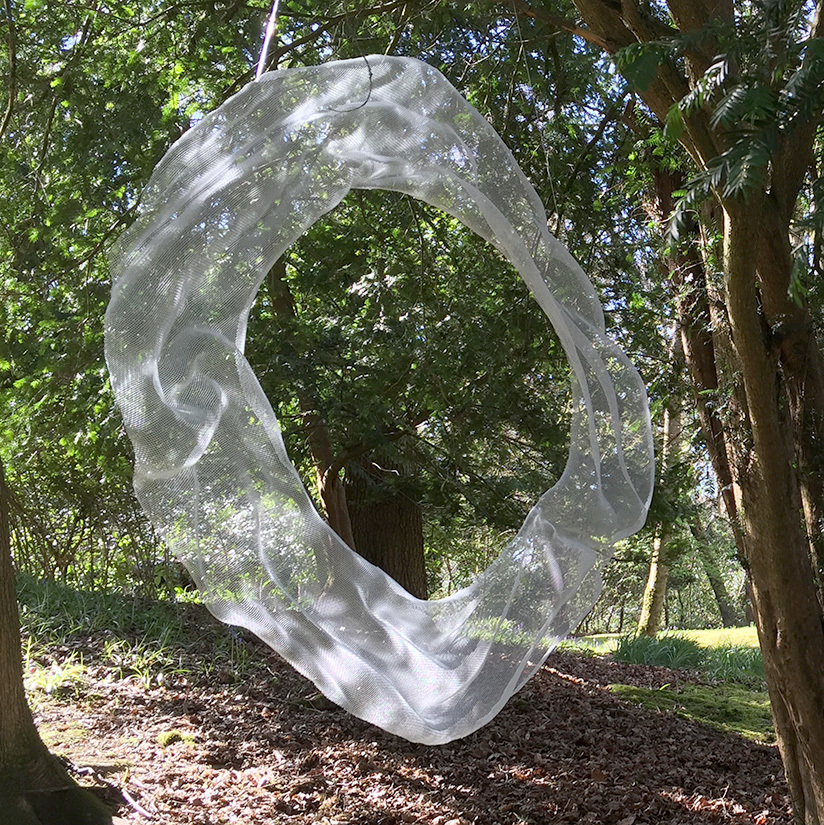Abstract bronzemesh sculpture
 /* styles */ Another anniversary and congratulations to Rachel Bebb for an excellent display of sculpture at the Garden Gallery, Hampshire for 25 years! For the new season the celebration and private view is on 1 June. On display are abstract sculpture ‘AWE‘ and figurative sculpture ‘BATHERS‘ which are both suspended bronzemesh sculptures with different colour finishes.
 table div table+table+table+table+table+table+table+table+table+table+table+table+table+table+table+table+table+table+table+table+table+table+table+table+table+table+table+table+table+table+table+table+table+table+table+table div table{width:100%;padding:0}table div table+table+table+table+table+table+table+table+table+table+table+table+table+table+table+table+table+table+table+table+table+table+table+table+table+table+table+table+table+table+table+table+table+table+table+table div table img{width:96.23%;padding:0;float:none}table div table+table+table+table+table+table+table+table+table+table+table+table+table+table+table+table+table+table+table+table+table+table+table+table+table+table+table+table+table+table+table+table+table+table+table+table div table td{width:100%;padding:0 1.88% 18px}/* styles */## JamHouse Birmingham: 20th Anniversary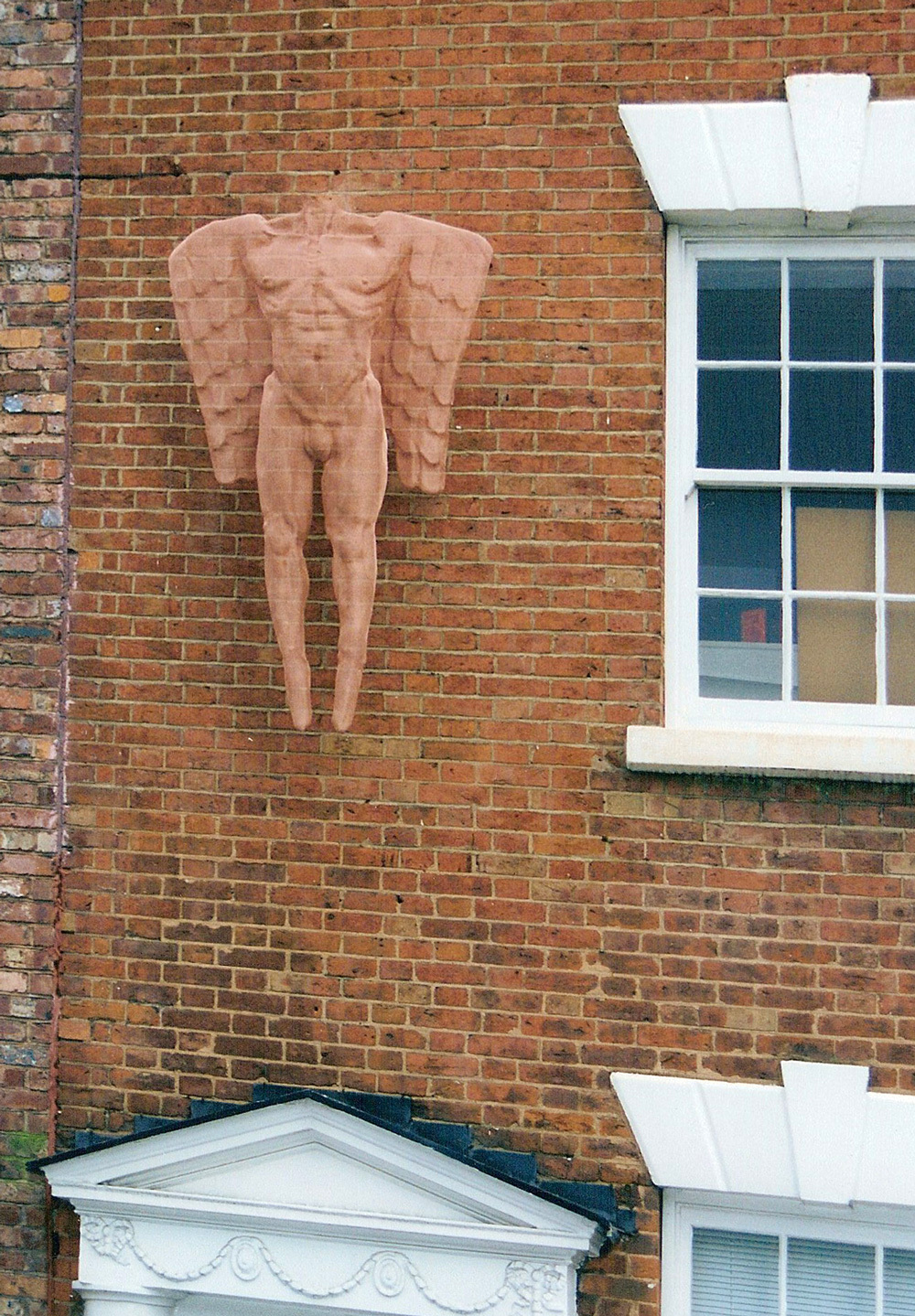‘MANANGEL‘ at the JamHouse Birmingham

JamHouse Birmingham celebrates 20 years of entertainment:
The JamHouse,
3-5 St Paul's Square,
Birmingham, B3 1QU.

20 years ago I was commissioned by Neil Tibbatts to create two large angel sculptures (ANANGEL and MANANGEL) for both inside and outside of the brand-new JamHouse, conceived by Neil in partnership with Jules Holland.

Some years later I was commissioned for a huge Buddha sculpture at Buddha-Bar, London, by Adam Tibbatts of Tibbatts Abel.

 table div table+table+table+table+table+table+table+table+table+table+table+table+table+table+table+table+table+table+table+table+table+table+table+table+table+table+table+table+table+table+table+table+table+table+table+table+table+table+table div table{width:100%;padding:0}table div table+table+table+table+table+table+table+table+table+table+table+table+table+table+table+table+table+table+table+table+table+table+table+table+table+table+table+table+table+table+table+table+table+table+table+table+table+table+table div table img{width:96.23%;padding:0;float:none}table div table+table+table+table+table+table+table+table+table+table+table+table+table+table+table+table+table+table+table+table+table+table+table+table+table+table+table+table+table+table+table+table+table+table+table+table+table+table+table div table td{width:100%;padding:0 1.88% 18px}/* styles */## With Easter coming up ...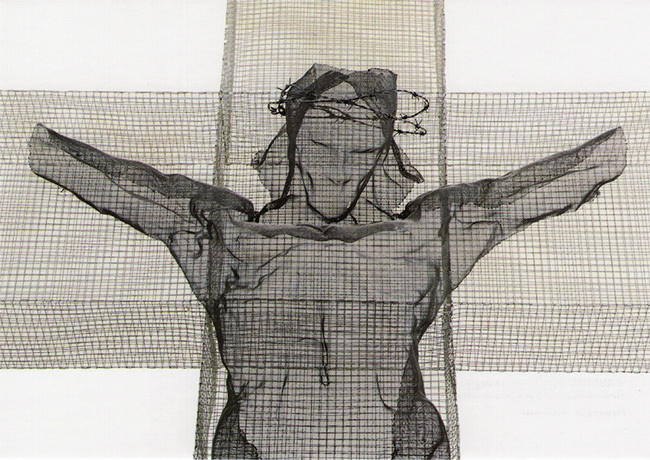Postcard ‘CRUCIFIX‘, photograph by Paul Brittain.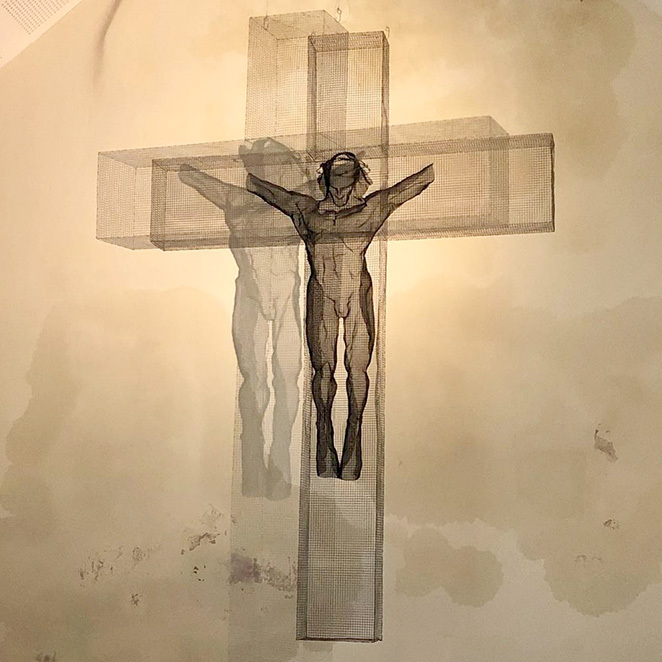Wire-Mesh Christ sculpture at the Barn Chapel, Shrine of Our Lady of Walsingham
 /* styles */ I was pleased to receive newly printed postcards depicting my sculpture ‘CRUCIFIX‘ 1988 (325 x 218 x 20 cm) located at the Barn Chapel, Shrine of Our Lady of Walsingham, Norfolk. The steel sculpture was created in 1988 for Winchester Cathedral and was later acquired for the Shrine in 2004. The arresting figure of Christ has no hands: "Christ has no hands but our hands" – derived from the prayer of S. Teresa of Avila. Here's a 2 page document (PDF) with information about this artwork to download.
 table div table+table+table+table+table+table+table+table+table+table+table+table+table+table+table+table+table+table+table+table+table+table+table+table+table+table+table+table+table+table+table+table+table+table+table+table+table+table+table+table+table+table+table div table{width:100%;padding:0}table div table+table+table+table+table+table+table+table+table+table+table+table+table+table+table+table+table+table+table+table+table+table+table+table+table+table+table+table+table+table+table+table+table+table+table+table+table+table+table+table+table+table+table div table img{width:96.23%;padding:0;float:none}table div table+table+table+table+table+table+table+table+table+table+table+table+table+table+table+table+table+table+table+table+table+table+table+table+table+table+table+table+table+table+table+table+table+table+table+table+table+table+table+table+table+table+table div table td{width:100%;padding:0 1.88% 18px}/* styles */## Violin Recital Concert

 table.module-44{width:69.43%;padding:0}table div table+table+table+table+table+table+table+table+table+table+table+table+table+table+table+table+table+table+table+table+table+table+table+table+table+table+table+table+table+table+table+table+table+table+table+table+table+table+table+table+table+table+table+table+table div table{width:69.43%;float:none;margin-left:auto;margin-right:auto;padding:0}table div table+table+table+table+table+table+table+table+table+table+table+table+table+table+table+table+table+table+table+table+table+table+table+table+table+table+table+table+table+table+table+table+table+table+table+table+table+table+table+table+table+table+table+table+table div table a{border:0 none;text-decoration:none}table div table+table+table+table+table+table+table+table+table+table+table+table+table+table+table+table+table+table+table+table+table+table+table+table+table+table+table+table+table+table+table+table+table+table+table+table+table+table+table+table+table+table+table+table+table div table img{width:100%!important;border:0 none;text-decoration:none}table div table+table+table+table+table+table+table+table+table+table+table+table+table+table+table+table+table+table+table+table+table+table+table+table+table+table+table+table+table+table+table+table+table+table+table+table+table+table+table+table+table+table+table+table+table div table td{width:100%;padding:0}/* styles */
 /* styles */ Finally here is an invitation to a very special performance at the Cadogan Hall in May, which is a Gala Fundraising Recital in the presence of patron HRH Princess Michael of Kent. Solo violonist Alda Dizdari will perform works by Debussy, Enescu, Ravel Elgar as a fund raiser for the upcoming recording of the Elgar Violin Concerto in Moscow. Please contact the Cadogan Hall for tickets and perhaps we'll see you there!
 table div table+table+table+table+table+table+table+table+table+table+table+table+table+table+table+table+table+table+table+table+table+table+table+table+table+table+table+table+table+table+table+table+table+table+table+table+table+table+table+table+table+table+table+table+table+table+table div table{width:100%;padding:0}table div table+table+table+table+table+table+table+table+table+table+table+table+table+table+table+table+table+table+table+table+table+table+table+table+table+table+table+table+table+table+table+table+table+table+table+table+table+table+table+table+table+table+table+table+table+table+table div table img{width:96.23%;padding:0;float:none}table div table+table+table+table+table+table+table+table+table+table+table+table+table+table+table+table+table+table+table+table+table+table+table+table+table+table+table+table+table+table+table+table+table+table+table+table+table+table+table+table+table+table+table+table+table+table+table div table td{width:100%;padding:0 1.88% 18px}/* styles */## Why do I receive this?

 /* styles */ We are writing to you as we understand you'd like to receive David Begbie's news from time to time. We hope no-one is receiving this mail-out who does not wish to. We are sharing news rarely - approx. 4 times a year and you can opt out anytime with the button below or by sending us an email. Many thanks for your continued interest!
 table div table+table+table+table+table+table+table+table+table+table+table+table+table+table+table+table+table+table+table+table+table+table+table+table+table+table+table+table+table+table+table+table+table+table+table+table+table+table+table+table+table+table+table+table+table+table+table+table+table+table+table div table{width:100%;padding:0}table div table+table+table+table+table+table+table+table+table+table+table+table+table+table+table+table+table+table+table+table+table+table+table+table+table+table+table+table+table+table+table+table+table+table+table+table+table+table+table+table+table+table+table+table+table+table+table+table+table+table+table div table img{width:96.23%;padding:0;float:none}table div table+table+table+table+table+table+table+table+table+table+table+table+table+table+table+table+table+table+table+table+table+table+table+table+table+table+table+table+table+table+table+table+table+table+table+table+table+table+table+table+table+table+table+table+table+table+table+table+table+table+table div table td{width:100%;padding:0 1.88% 18px}/* styles */table div table+table+table+table+table+table+table+table+table+table+table+table+table+table+table+table+table+table+table+table+table+table+table+table+table+table+table+table+table+table+table+table+table+table+table+table+table+table+table+table+table+table+table+table+table+table+table+table+table+table+table+table div table,table.module-51{width:64.53%;float:right;padding:0}table div table+table+table+table+table+table+table+table+table+table+table+table+table+table+table+table+table+table+table+table+table+table+table+table+table+table+table+table+table+table+table+table+table+table+table+table+table+table+table+table+table+table+table+table+table+table+table+table+table+table+table+table div table a{border:0 none;text-decoration:none}table div table+table+table+table+table+table+table+table+table+table+table+table+table+table+table+table+table+table+table+table+table+table+table+table+table+table+table+table+table+table+table+table+table+table+table+table+table+table+table+table+table+table+table+table+table+table+table+table+table+table+table+table div table img{width:100%!important;border:0 none;text-decoration:none}table div table+table+table+table+table+table+table+table+table+table+table+table+table+table+table+table+table+table+table+table+table+table+table+table+table+table+table+table+table+table+table+table+table+table+table+table+table+table+table+table+table+table+table+table+table+table+table+table+table+table+table+table div table td{width:100%;padding:0 0 20px 20px}/* styles */ Wiremesh kindly provided by United Wire, Edinburgh